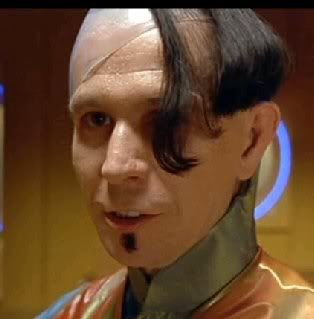###Author Topic: Problem 3  (Read 7188 times)

#### Ian Kivlichan

• Sr. Member
•• Posts: 51
• Karma: 17##### Problem 3
« on: December 20, 2012, 01:32:15 PM »
Use separation of variables to solve the Dirichlet problem for the Laplacian on the unit disk $\mathbb{D} = \{ (x,y) \in \mathbb{R}^2: x^2 + y^2 < 1\}$ with boundary condition $u(1, \theta) = \cos \theta.$
(The boundary condition is described in polar coordinates $(r, \theta) \rightarrow u(r, \theta)$ along $r=1$).

hopeful solution attached! (since djirar is posting all the solutions right away after 13:30..)
« Last Edit: December 20, 2012, 01:49:28 PM by Victor Ivrii »

#### Chen Ge Qu

• Full Member
•• Posts: 16
• Karma: 8##### Re: Problem 3
« Reply #1 on: December 20, 2012, 01:36:38 PM »
Yeah, I thought we were supposed to wait for Prof. Ivrii to post problems as well, but my solution to 3 is attached!

#### Ian Kivlichan

• Sr. Member
•• Posts: 51
• Karma: 17##### Re: Problem 3
« Reply #2 on: December 20, 2012, 02:26:11 PM »
Chen Ge: I would point out that the solutions to the Euler equation are $R(r) = Ar^n + Br^{-n}$, not $R(r) = Ar^n + \frac{B}{r^{n+1}}$. It makes no difference, but I think it is worth pointing out anyway.

#### Pei Zhou

• Newbie
•• Posts: 4
• Karma: 3##### Re: Problem 3
« Reply #3 on: December 20, 2012, 04:50:46 PM »

#### Victor Ivrii##### Re: Problem 3
« Reply #4 on: December 23, 2012, 04:35:43 AM »
I started to grade Problem 3 and as Zorg "I am very disappointed" (but trying to be very generous).• Really smart solution: $u = \cos (\theta)= r\cos(\theta)=x$ as $r=1$ but $x$ is a harmonic function so $\boxed{u=x}$.
• More industrious solution: Starting separation of variables and arrive to $\cos(n\theta)$ and $\sin(n\theta)$ and from boundary condition we need to consider only $\cos(\theta)$, so $u=(Ar+Br^{-1})\cos(\theta)$ and to exclude singularity as $r=0$ we have $B=0$, then $u=Ar\cos(\theta)$ and from the boundary condition $A=1$ and $\boxed{u=r\cos(\theta)}$.
• Industrious solution: Applying separation of variables and excluding terms singular  as $r=0$ we arrive to
\begin{equation}
u=\frac{1}{2}A_0+\sum_{n=1}^\infty r^n\bigl(A_n\cos(n\theta)+B_n\sin(n\theta)\bigr);
\label{eq-1}
\end{equation}
plugging into boundary condition we get
\begin{equation}
\cos(\theta)=\frac{1}{2}A_0+\sum_{n=1}^\infty \bigl(A_n\cos(n\theta)+B_n\sin(n\theta)\bigr);
\label{eq-2}
\end{equation}
then obviously $B_n=0$ for all $n$ while $A_1=1$ and $A_n=0$ for all $n\ne 1$ (the last attempt to be smart!) and $\boxed{u=r\cos(\theta)}$.
• Really industrious solution: Calculate $A_n$ and $B_n$ by formulae and find out that   $B_n=0$ for all $n$ while $A_1=1$ and $A_n=0$ for all $n\ne 1$ and again $\boxed{u=r\cos(\theta)}$.
• Many started however calculate $A_n$ and $B_n$ and got wrong answers! Even a Wise Man Stumbles.
« Last Edit: December 23, 2012, 04:37:58 AM by Victor Ivrii »

#### Victor Ivrii##### Re: Problem 3
« Reply #5 on: December 23, 2012, 05:02:09 AM »
• BTW, writing in general
\begin{equation}
u=\frac{1}{2}(A_0+B_0\log r)+\sum_{n=1}^\infty \bigl(c_nr^n+d_nr^{-n}\bigr)\bigl(a_n\cos(n\theta)+b_n\sin(n\theta)\bigr)
\label{eq-3}
\end{equation}
is an error. Correct general answer is
\begin{equation}
u=\frac{1}{2}(A_0+B_0\log r)+\sum_{n=1}^\infty \bigl[ r^n  \bigl(A_n\cos(n\theta)+B_n\sin(n\theta)\bigr)+r^{-n} \bigl(C_n\cos(n\theta)+D_n\sin(n\theta)\bigr)\bigr].
\label{eq-4}
\end{equation}
What the difference?  While (\ref{eq-4}) contains 4 independent coefficients, (\ref{eq-3}) contains only 3 because $A_n=c_na_n, B_n=c_nb_n, C_n=d_na_n, D_n=d_nb_n$ satisfy $A_nD_n=B_nC_n$.
• Periodicity condition $\Phi(0)=\Phi(2\pi)$, $\Phi'(0)=\Phi'(2\pi)$.
$\color{red}{\Phi(0)=\Phi(2\pi)=0}$--WRONG!  (etc)
One should get full Fourier series--with both $\cos(n\theta)$ and $\sin(n\theta)$.
« Last Edit: December 23, 2012, 11:24:00 AM by Victor Ivrii »